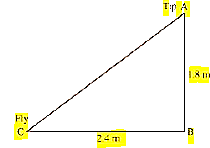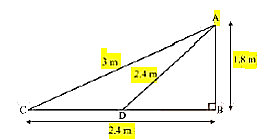Guru

# Nazima is fly fishing in a stream. The tip of her fishing rod is 1.8 m above the surface of the water and the fly at the end of the string rests on the water 3.6 m away and 2.4 m from a point directly under the tip of the rod. Assuming that her string (from the tip of her rod to the fly) is taut, how much string does she have out (see Figure)? If she pulls in the string at the rate of 5 cm per second, what will be the horizontal distance of the fly from her after 12 seconds? Q.10

• 0

How i solve this problem in easy way of Triangles chapter of exercise 6.6 of ncert math of last question of optional exercise, give me the best and easy way to solve this ncert question Nazima is fly fishing in a stream. The tip of her fishing rod is 1.8 m above the surface of the water and the fly at the end of the string rests on the water 3.6 m away and 2.4 m from a point directly under the tip of the rod. Assuming that her string (from the tip of her rod to the fly) is taut, how much string does she have out (see Figure)? If she pulls in the string at the rate of 5 cm per second, what will be the horizontal distance of the fly from her after 12 seconds?

Share

1. Let us consider, AB is the height of the tip of the fishing rod from the water surface and BC is the

horizontal distance of the fly from the tip of the fishing rod. Therefore, AC is now the length of the string.To find AC, we have to use Pythagoras theorem in ∆ABC, is such way;

AC2 = AB2+ BC2

AB2 = (1.8 m) 2 + (2.4 m) 2

AB2 = (3.24 + 5.76) m2

AB2 = 9.00 m2

⟹ AB = √9 m = 3m

Thus, the length of the string out is 3 m.

As its given, she pulls the string at the rate of 5 cm per second.

Therefore, string pulled in 12 seconds = 12 × 5 = 60 cm = 0.6 mLet us say now, the fly is at point D after 12 seconds.

Length of string out after 12 seconds is AD.

AD = AC − String pulled by Nazima in 12 seconds

= (3.00 − 0.6) m

= 2.4 m

In ∆ADB, by Pythagoras Theorem,

AB2 + BD2 = AD2

(1.8 m) 2 + BD2 = (2.4 m) 2

BD2 = (5.76 − 3.24) m2 = 2.52 m2

BD = 1.587 m

Horizontal distance of fly = BD + 1.2 m

= (1.587 + 1.2) m = 2.787 m

= 2.79 m

• 0